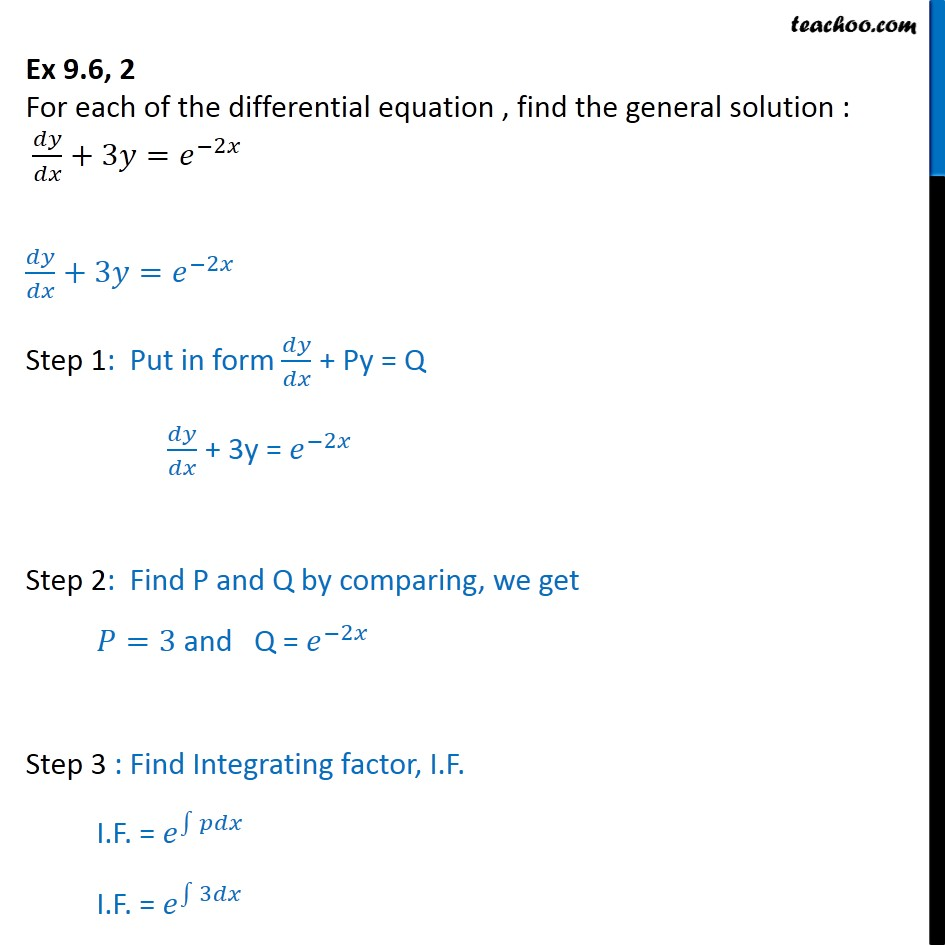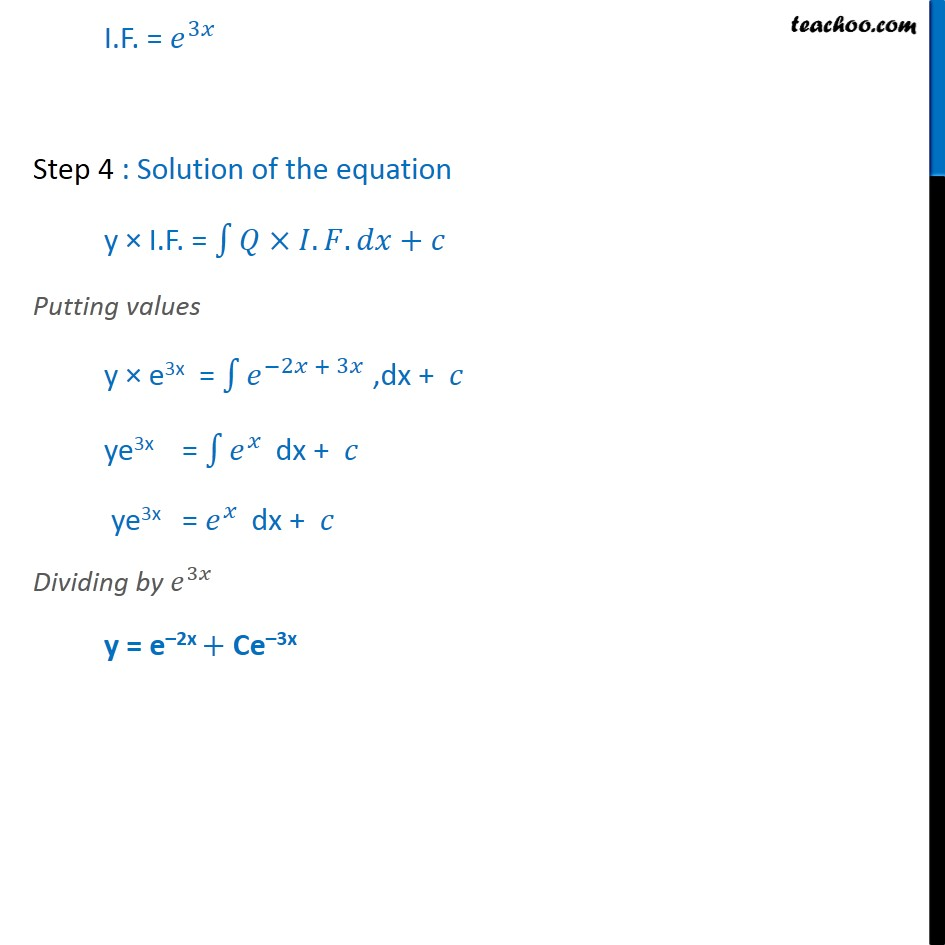Ex 9.5

Chapter 9 Class 12 Differential Equations
Serial order wiseLearn in your speed, with individual attention - Teachoo Maths 1-on-1 Class

### Transcript

Ex 9.5, 2 For each of the differential equation , find the general solution : 𝑑𝑦﷮𝑑𝑥﷯+3𝑦= 𝑒﷮−2𝑥﷯ 𝑑𝑦﷮𝑑𝑥﷯+3𝑦= 𝑒﷮−2𝑥﷯ Step 1: Put in form 𝑑𝑦﷮𝑑𝑥﷯ + Py = Q 𝑑𝑦﷮𝑑𝑥﷯ + 3y = 𝑒﷮−2𝑥﷯ Step 2: Find P and Q by comparing, we get 𝑃=3 and Q = 𝑒﷮−2𝑥﷯ Step 3 : Find Integrating factor, I.F. I.F. = 𝑒﷮ ﷮﷮𝑝𝑑𝑥﷯﷯ I.F. = 𝑒﷮ ﷮﷮3𝑑𝑥﷯﷯ I.F. = 𝑒﷮3𝑥﷯ Step 4 : Solution of the equation y × I.F. = ﷮﷮𝑄×𝐼.𝐹. 𝑑𝑥+𝑐﷯ Putting values y × e3x = ﷮﷮ 𝑒﷮−2𝑥 + 3𝑥﷯﷯ ,dx + 𝑐 ye3x = ﷮﷮ 𝑒﷮𝑥 ﷯﷯ dx + 𝑐 ye3x = 𝑒﷮𝑥 ﷯ dx + 𝑐 Dividing by 𝑒﷮3𝑥 ﷯ y = e–2x + Ce–3x